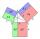The pond

We can see the pond at an angle 65°37'. Its end points are 155 m and 177 m away from the observer. What is the width of the pond?

Result

c =  180.836 m

Solution:Try calculation via our triangle calculator.

Leave us a comment of example and its solution (i.e. if it is still somewhat unclear...):Be the first to comment!To solve this example are needed these knowledge from mathematics:

Pythagorean theorem is the base for the right triangle calculator. Cosine rule uses trigonometric SAS triangle calculator. See also our trigonometric triangle calculator.

Next similar examples:

1. Triangle ABCIn a triangle ABC with the side BC of length 2 cm The middle point of AB. Points L and M split AC side into three equal lines. KLM is isosceles triangle with a right angle at the point K. Determine the lengths of the sides AB, AC triangle ABC.
2. Triangle ABCTriangle ABC has side lengths m-1, m-2, m-3. What has to be m to be triangle a) rectangular b) acute-angled?
3. Medians of isosceles triangleThe isosceles triangle has a base ABC |AB| = 16 cm and 10 cm long arm. What are the length of medians?
4. Angles by cosine lawCalculate the size of the angles of the triangle ABC, if it is given by: a = 3 cm; b = 5 cm; c = 7 cm (use the sine and cosine theorem).
5. If theIf the tangent of an angle of a right angled triangle is 0.8. Then its longest side is. .. .
6. Euclid2In right triangle ABC with right angle at C is given side a=27 and height v=12. Calculate the perimeter of the triangle.
7. Scalene triangleSolve the triangle: A = 50°, b = 13, c = 6
8. Side cIn △ABC a=2, b=4 and ∠C=100°. Calculate length of the side c.
9. Greatest angleCalculate the greatest triangle angle with sides 197, 208, 299.
10. Circle annulusThere are 2 concentric circles in the figure. Chord of larger circle 10 cm long is tangent to the smaller circle. What are does annulus have?
11. Theorem proveWe want to prove the sentence: If the natural number n is divisible by six, then n is divisible by three. From what assumption we started?
12. CalculateCalculate the length of a side of the equilateral triangle with an area of 50cm2.
13. LawsFrom which law follows directly the validity of Pythagoras' theorem in the right triangle? ?
14. Isosceles IVIn an isosceles triangle ABC is |AC| = |BC| = 13 and |AB| = 10. Calculate the radius of the inscribed (r) and described (R) circle.
15. Triangle and its heightsCalculate the length of the sides of the triangle ABC, if va=5 cm, vb=7 cm and side b is 5 cm shorter than side a.
16. Vector 7Given vector OA(12,16) and vector OB(4,1). Find vector AB and vector |A|.
17. ABS CNCalculate the absolute value of complex number -15-29i.